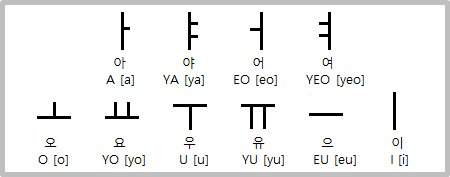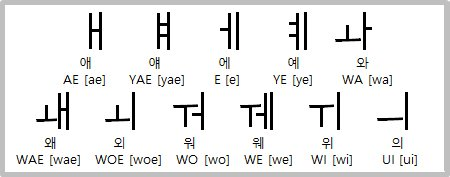### Learn Korean – The Basics Part 2!

Posted by : AsmaLet’s get started with Part 2!

Earlier in part-1 we went through the 14 consonants and their twin counterparts in Korean. In this part we’ll be looking at the 10 vowels as well as the combined vowels, and also try to make some words using the consonants and vowels that we’ve learnt.BASIC VOWELS – Like it was mentioned above, there are 10 basic vowels in the Korean language. They are – ㅏ ㅑ ㅓ ㅕ ㅗ ㅛ ㅜ ㅠ ㅡ ㅣ. Fun Fact – The symbols of Heaven (.),  Earth ( ), and Man (ㅣ) are used to make up the vowels in Korean, do note that the dot symbolizing Heaven is now often written as a short horizontal line instead of the dot. For example – which is the first vowel is a combination of Man (ㅣ) and Heaven (.) and is pronounced as “Ah..”

Let’s look at the sound of the vowels.

•  has the sound of “a” – as in the word Car.
• has the sound of “ya” – as in the word See ya.
• has the sound of “eo” – as in the word Under.
• has the sound of “yeo” – as in the word Young.
• ㅗ has the sound of “o” – as in the word Orange.
• ㅛ has the sound of “yo” – as in the word Yoyo.
• ㅜ has the sound of “u” – as in the word Boon.
• ㅠ has the sound of “yu” – as in the word You.
• ㅡ has the sound of “eu” – There isn’t exactly a sound of this vowel in English so it’s difficult to list down a word. I could only think of the word Good to being the closest in the “eu” sound.
• has the sound of “i” – as in the word Ski.COMBINED VOWELS – The basic vowels are put together to create combined vowel sounds, also known as dipthongs. Once you’ve mastered the basic vowels, you’ll be able to understand the combined vowels in no time. Some of these sounds very similar to each other so don’t get stressed if you can’t distinguish the sounds (Some native Koreans find it difficult to distinguish the sounds as well). The combined vowels are – ㅐ,ㅒ, ㅔ, ㅖ, ㅘ, ㅙ, ㅚ, ㅝ, ㅞ, ㅟ, ㅢ.

Let’s look at the sounds of these vowels –

• has the sound of “ae” and is a combination of (a) +(i).
• has the sound of “yae” and is a combination of(a) +(eo).
• has the sound of “e” and is a combination of (eo) +(i) – The romanizations of ㅒandㅔis different but they sound pretty similar to each other.
• has the sound of “ye” and is a combination of (yeo) + (i).
• ㅘ has the sound of “wa” and is the combination of ㅗ (o) + (a).
• ㅙ has the sound of “wae” and is the combination of ㅗ (o) + (ae).
• ㅚ has the sound of “woe” and is the combination of  ㅗ (o) + (i).
• ㅝ has the sound of “wo” and is the combination of  ㅜ(u) + (eo).
• ㅞ has the sound of “we” and is the combination of (u) + (e).
• ㅟ has the sound of “wi” and is the combination of (u) + (i).
• ㅢ has the sound of “ui” and is the combination of (eu) + (i).

So now that we’ve finished learning the vowels and consonants, should we try combining them and make words?

ㅍ +ㅣ+ㅈ+ㅏ = 피자 (Pizza)

ㅅ +ㅏ+ㄱ+ㅘ = 사과 (Apple)

ㅇ+ ㅗ+ ㄹ+ㅔ+ㄴ+ㅈ+ㅣ= 오렌지 (Orange)

ㅌ+ㅗ+ㅁ+ㅏ+ㅌ+ㅗ = 토마토 (Tomato)

Once you know the consonants and vowels well, you’ll be able to make words easily. So, how was today’s lesson? Was it less confusing than the consonants or more because of the similar pronunciations? I’ll be back with another lesson tomorrow. Till then, Happy Learning!